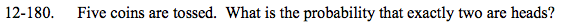### Home > A2C > Chapter 12 > Lesson 12.4.2 > Problem12-180

12-180.

Five coins are tossed. What is the probability that exactly two are heads? Homework Help ✎Since the tosses are independent, how many total outcomes could there be in 5 tosses?

2 · 2 · 2 · 2 · 2 · = 32

Order does not matter, use 5C2.

5C2 = 10

$\frac{10}{32}=\frac{5}{16}$